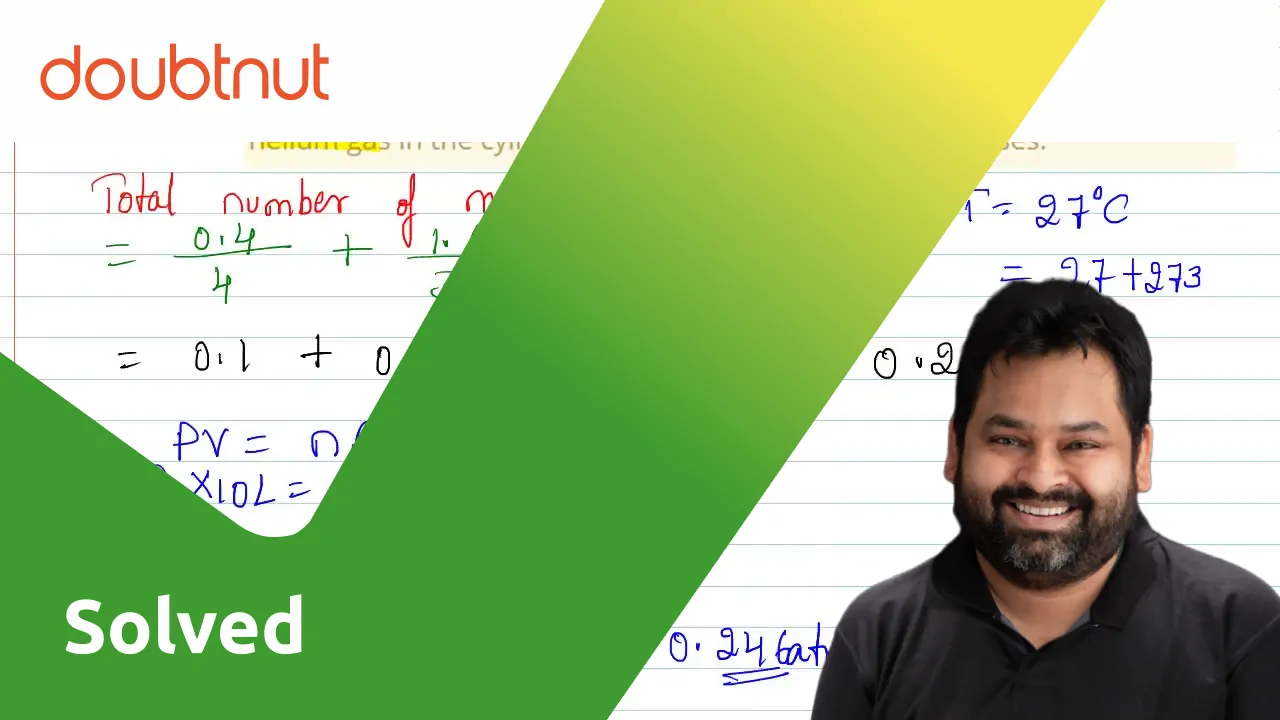Doubtnut App - Get 50 Lakh+ video solutions better than GoogleInstall Now

# Calculate the total pressure in a 10 L cylinder which contains 0.4 g helium ,1.6 of oxygen and 1.4 g nitrogen at 27∘C. Also calculate the partial of helium gas in the cylinder. Assume ideal hehaviour for gases. Solution in Kannada

Text Solution
Verified by Experts

## Total number of moles =0⋅44+1⋅632+1⋅428=0.1+05+0⋅05=0⋅2 moles. pV=nRT p×10L=0⋅2×0⋅82×300⇒p=24⋅6×0⋅210 p=0⋅492atm PHe0.10.2×0⋅492=0⋅246 atm